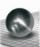# Hemispherical hollow

The vessel hemispherical hollow is filled with water to a height of 10 cm =. How many liters of water are inside if the inside diameter of the hollow is d = 28cm?

Result

V =  3.351 l

#### Solution:Leave us a comment of example and its solution (i.e. if it is still somewhat unclear...):

Showing 0 comments:Be the first to comment!## Next similar examples:

1. Hollow sphereThe volume of the hollow ball is 3432 cm3. What is its internal radius when the wall thickness is 3 cm?
2. GasholderThe gasholder has spherical shape with a diameter 20 m. How many m3 can hold in?
3. Three unknownsSolve the system of linear equations with three unknowns: A + B + C = 14 B - A - C = 4 2A - B + C = 0
4. TetrahedronCalculate height and volume of a regular tetrahedron whose edge has a length 18 cm.
5. Cube cornersFrom cube of edge 14 cm cut off all vertices so that each cutting plane intersects the edges 1 cm from the nearest vertice. How many edges will have this body?
6. ConfectioneryThe village markets have 5 kinds of sweets, one weighs 31 grams. How many different ways a customer can buy 1.519 kg sweets.
7. TeamsHow many ways can divide 16 players into two teams of 8 member?
8. BlocksThere are 9 interactive basic building blocks of an organization. How many two-blocks combinations are there?
9. Theorem proveWe want to prove the sentense: If the natural number n is divisible by six, then n is divisible by three. From what assumption we started?
10. LegsCancer has 5 pairs of legs. The insect has 6 legs. 60 animals have a total of 500 legs. How much more are cancers than insects?
11. AP - simpleDetermine the first nine elements of sequence if a10 = -1 and d = 4
12. Elimination methodSolve system of linear equations by elimination method: 5/2x + 3/5y= 4/15 1/2x + 2/5y= 2/15
13. TrigonometryIs true equality? ?
14. LineIt is true that the lines that do not intersect are parallel?
15. Sequence 2Write the first 5 members of an arithmetic sequence a11=-14, d=-1
16. SequenceWrite the first 6 members of these sequence: a1 = 5 a2 = 7 an+2 = an+1 +2 an
17. AverageIf the average(arithmetic mean) of three numbers x,y,z is 50. What is the average of there numbers (3x +10), (3y +10), (3z+10) ?